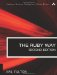# Section 3.8. Using Character Classes

### 3.7. Accessing Backreferences

Each parenthesized piece of a regular expression will be a submatch of its own. These are numbered and can be referenced by these numbers in more than one way. Let's examine the more traditional "ugly" ways first.

The special global variables \$1, \$2, and so on, can be used to reference matches:

`str = "a123b45c678" if /(a\d+)(b\d+)(c\d+)/ =~ str   puts "Matches are: '#\$1', '#\$2', '#\$3'"   # Prints: Matches are: 'a123', 'b45', 'c768' end`

Within a substitution such as sub or gsub, these variables cannot be used:

`str = "a123b45c678" str.sub(/(a\d+)(b\d+)(c\d+)/, "1st=#\$1, 2nd=#\$2, 3rd=#\$3") # "1st=, 2nd=, 3rd="`

Why didn't this work? Because the arguments to sub are evaluated before sub is called. This code is equivalent:

`str = "a123b45c678" s2 = "1st=#\$1, 2nd=#\$2, 3rd=#\$3" reg = /(a\d+)(b\d+)(c\d+)/ str.sub(reg,s2) # "1st=, 2nd=, 3rd="`

This code, of course, makes it much clearer that the values \$1 through \$3 are unrelated to the match done inside the sub call.

In this kind of case, the special codes \1, \2, and so on, can be used:

`str = "a123b45c678" str.sub(/(a\d+)(b\d+)(c\d+)/, '1st=\1, 2nd=\2, 3rd=\3') # "1st=a123, 2nd=b45, 3rd=c768"`

Notice that we used single quotes (hard quotes) in the preceding example. If we used double quotes (soft quotes) in a straightforward way, the backslashed items would be interpreted as octal escape sequences:

`str = "a123b45c678" str.sub(/(a\d+)(b\d+)(c\d+)/, "1st=\1, 2nd=\2, 3rd=\3") # "1st=\001, 2nd=\002, 3rd=\003"`

The way around this is to double-escape:

`str = "a123b45c678" str.sub(/(a\d+)(b\d+)(c\d+)/, "1st=\\1, 2nd=\\2, 3rd=\\3") # "1st=a123, 2nd=b45, 3rd=c678"`

It's also possible to use the block form of a substitution, in which case the global variables may be used:

`str = "a123b45c678" str.sub(/(a\d+)(b\d+)(c\d+)/)  { "1st=#\$1, 2nd=#\$2, 3rd=#\$3" } # "1st=a123, 2nd=b45, 3rd=c678"`

When using a block in this way, it is not possible to use the special backslashed numbers inside a double-quoted string (or even a single-quoted one). This is reasonable if you think about it.

As an aside here, I will mention the possibility of noncapturing groups. Sometimes you may want to regard characters as a group for purposes of crafting a regular expression; but you may not need to capture the matched value for later use. In such a case, you can use a noncapturing group, denoted by the (?:...) syntax:

`str = "a123b45c678" str.sub(/(a\d+)(?:b\d+)(c\d+)/, "1st=\\1, 2nd=\\2, 3rd=\\3") # "1st=a123, 2nd=c678, 3rd="`

In the preceding example, the second grouping was thrown away, and what was the third submatch became the second.

I personally don't like either the \1 or the \$1 notations. They are convenient sometimes, but it isn't ever necessary to use them. We can do it in a "prettier," more object-oriented way.

The class method Regexp.last_match returns an object of class MatchData (as does the instance method match). This object has instance methods that enable the programmer to access backreferences.

The MatchData object is manipulated with a bracket notation as though it were an array of matches. The special element 0 contains the text of the entire matched string. Thereafter, element n refers to the nth match:

`pat = /(.+[aiu])(.+[aiu])(.+[aiu])(.+[aiu])/i # Four identical groups in this pattern refs = pat.match("Fujiyama") # refs is now: ["Fujiyama","Fu","ji","ya","ma"] x = refs y = refs[2..3] refs.to_a.each {|x| print "#{x}\n"}`

Note that the object refs is not a true array. Thus when we want to treat it as one by using the iterator each, we must use to_a (as shown) to convert it to an array.

We may use more than one technique to locate a matched substring within the original string. The methods begin and end return the beginning and ending offsets of a match. (It is important to realize that the ending offset is really the index of the next character after the match.)

`str = "alpha beta gamma delta epsilon" #      0....5....0....5....0....5.... #      (for  your counting convenience) pat = /(b[^ ]+ )(g[^ ]+ )(d[^ ]+ )/ # Three words, each one a single match refs = pat.match(str) # "beta " p1 = refs.begin(1)         # 6 p2 = refs.end(1)           # 11 # "gamma " p3 = refs.begin(2)         # 11 p4 = refs.end(2)           # 17 # "delta " p5 = refs.begin(3)         # 17 p6 = refs.end(3)           # 23 # "beta gamma delta" p7 = refs.begin(0)         # 6 p8 = refs.end(0)           # 23`

Similarly, the offset method returns an array of two numbers, which are the beginning and ending offsets of that match. To continue the previous example:

`range0 = refs.offset(0)    # [6,23] range1 = refs.offset(1)    # [6,11] range2 = refs.offset(2)    # [11,17] range3 = refs.offset(3)    # [17,23]`

The portions of the string before and after the matched substring can be retrieved by the instance methods pre_match and post_match, respectively. To continue the previous example:

`before = refs.pre_match    # "alpha " after  = refs.post_match   # "epsilon"`The Ruby Way, Second Edition: Solutions and Techniques in Ruby Programming (2nd Edition)
ISBN: 0672328844
EAN: 2147483647
Year: 2004
Pages: 269
Authors: Hal Fulton

Similar book on Amazon

flylib.com © 2008-2017.
If you may any questions please contact us: flylib@qtcs.net